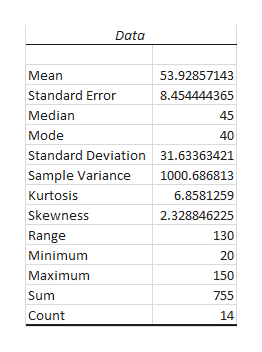# A survey of the mean number of cents off that coupons give was conducted by randomly surveying one coupon per page from the coupon sections of a recent San Jose Mercury News. The following data were collected: 20¢; 75¢; 50¢; 65¢; 30¢; 55¢; 40¢; 40¢; 30¢; 55¢; \$1.50; 40¢; 65¢; 40¢. Assume the underlying distribution is approximately normal.Construct a 95% confidence interval for the population mean worth of coupons .What is the lower bound? ( Round to 3 decimal places )What is the upper bound? ( Round to 3 decimal places )What is the error bound? (Round to 3 decimal places)

Question
76 views

A survey of the mean number of cents off that coupons give was conducted by randomly surveying one coupon per page from the coupon sections of a recent San Jose Mercury News. The following data were collected: 20¢; 75¢; 50¢; 65¢; 30¢; 55¢; 40¢; 40¢; 30¢; 55¢; \$1.50; 40¢; 65¢; 40¢. Assume the underlying distribution is approximately normal.

Construct a 95% confidence interval for the population mean worth of coupons .

What is the lower bound? ( Round to 3 decimal places )

What is the upper bound? ( Round to 3 decimal places )

What is the error bound? (Round to 3 decimal places)

check_circle

star
star
star
star
star
1 Rating
Step 1

From the given data, one of the data value is in dollars. So, first convert it into cents. That is, \$1.50 = 150 cents.

Step-by-step procedure to find sample mean, standard deviation and sample size using Excel:

• In Excel sheet, enter Data in one column.
• In Data, select Data Analysis and Choose Descriptive Statistics.
• In Input Range, select Data and click Labels in First Row.
• Select Summary Statistics.
• Click OK.

Output using Excel is shown below:help_outlineImage TranscriptioncloseData Mean 53.92857143 Standard Error 8.454444365 Median 45 Mode 40 Standard Deviation 31.63363421 Sample Variance 1000.686813 Kurtosis 6.8581259 Skewness 2.328846225 Range 130 Minimum 20 Maximum 150 Sum 755 Count 14 fullscreen
Step 2

From the output, the sample mean, standard deviation and sample size are 53.93, 31.63 and 14, respectively.

The formula for finding confidence interval for population mean is shown below:

Step 3

For 95% confidence level, the value of tα/2 = t0.05/2 at 13 (=14–1) degrees of freedom using t ...

### Want to see the full answer?

See Solution

#### Want to see this answer and more?

Solutions are written by subject experts who are available 24/7. Questions are typically answered within 1 hour.*

See Solution
*Response times may vary by subject and question.
Tagged in

### Statistics